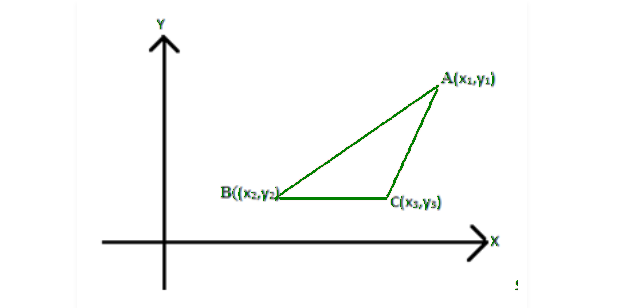Open in App
Not now

# Area of a Triangle using Determinants | Class 12 Maths

• Last Updated : 27 Nov, 2020

We have previously learned that the determinant is the scalar value which is computed from different elements of the square matrix that has certain properties of a linear transformation. Let us now learn how to use the determinants to find the area of the triangle, let’s say that (x1, y1), (x2, y2), (x3, y3) are the 3 points of the triangle in the Cartesian plane now the area of the triangle will be given as

### Formula for the Area of Trianglewhere,

∝ = Area of Triangle

x1, y1, x2, y2, x3, and y3 = Vertices of triangleThe formula for locating area might be represented within the sort of determinants as given below:As we all know the worth of a determinant can either be negative or a positive value but since we are talking about an area and it can never be taken as a negative value, therefore we take absolutely the value of the determinant so obtained.

If the world of Triangulum is already given then we make use of both the positive and negative values of the determinant.

Also, if three points are collinear we might be left with a line rather than a triangle and because the area enclosed by a line is zero hence the value of the determinant will also be zero.

Keeping the above-mentioned points in mind, allow us to attempt to expand the determinant which denoted the world by using determinant expansion techniques using minors and cofactors.

### Problems based on the area of atriangle

Problem 1: Find the area of the triangle whose vertices are (0, 0), (1, 2), and (4, 3).

Solution:

Let the point be (x1, y1)  ==>  (0, 0), (x2, y2) ==> (1, 2) and (x3, y3) ==> (4, 3)= (1/2)[3 – 8]

= (1/2)[-5]

= -5/2

= -2.5

Area cannot be represented with negative. Hence, area of the triangle with given vertices is 2.5 square units.

Problem 2: If (k, 2), (2, 4) and (3, 2) are vertices of the triangle of area 4 square units then determine the value of k.

Solution:

Area of triangle  = 4 square units(1/2){k [4 – 2] – 2[2 – 3] + 1[4 – 12]} = 4

k(2) – 2(-1) + 1(-8) = 8

2k + 2 – 8 = 8

2k – 6 = 8

2k = 8 + 6

2k = 14

k = 7

So, the value of k is 7.

Problem 3: Vertices of a triangle are (-2, -3), (3, 2), and (-1, -8). Find the area of triangle??

Solution:= (1/2) [ -2(2 + 8) + 3(3+1) + 1(-24 + 2) ]

= (1/2) [-2 (10) + 3(4) + 1(-22)]

= (1/2) [ -20 + 12 – 22]

= (1/2) [ -42 + 12]

= (1/2) (-30)

= -15

So, the required area is 15 square units.

My Personal Notes arrow_drop_up
Related Articles